+ +

# Laws of reflection on mirrors

Know some laws of reflection of light on spherical curved mirrors is useful to understand the basic technologies such as corneal topography specular, and understand the genesis of the light reflections that can be seen when one illuminates the eye, including the easiest to observe is the reflection corneal, also called image of Purkinje.

As for the laws of refraction, sought to resolve issues the position of the plan that forms the image (reflection) and the size of this image (its magnification).

The eye against the light path three surfaces that act as convex mirrors: the anterior face of the cornea, the posterior side of the cornea, the front of the lens. Only a surface acts like a concave mirror: the posterior side of the lens. The percentage of the reflected incident light is close to 5% for the front of the cornea, and much lower for images formed by reflection on the other surfaces.

Under the Huygens principle and time of minimum course imposed on the path of light, reflection of a beam on a flat surface is not a problem. The angle of impact with the surface (where the normal point of impact) equals the reflected angle.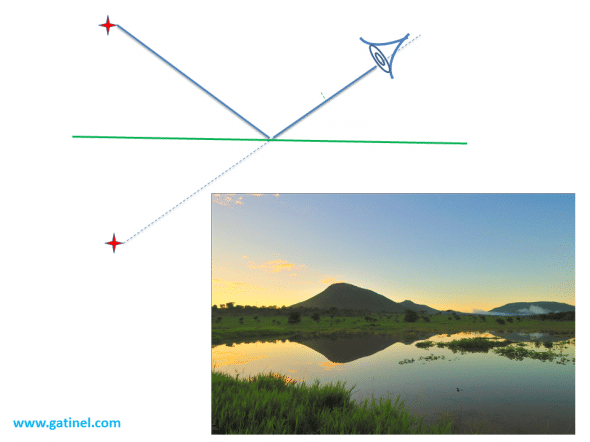Reflection plane (top). The reflected image undergoes a reversal. The eye watching the mirror in a given direction fixed point of the image such as the time set by the light from its source until the eye is minimal (equivalent to the 'straight line' ride. (down example of reflection hovers in the wild)

An incident Ray on the smooth surface of a curved mirror forms an angle with the normal to the surface (which can be regarded as locally flat). It is thought an identical angle to this normal. In the case of mirrors curved, circular in section, the normal to the surface marries the path of the local radius of curvature of this section.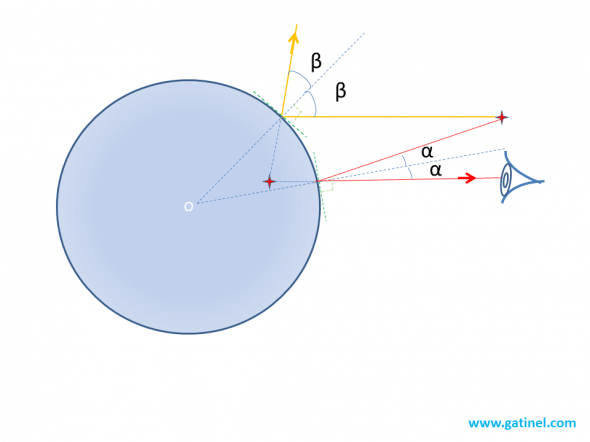The observer and the source are not aligned with the center of the mirror: the light rays that reach the observer are reflected according to an equal angle on part and on the other of the normal to the surface at the point of reflection.

## Concave mirror

Only the posterior side of the lens (or implant) behaves like a concave mirror. We focus on a simple system, a circular concave mirror where a close incident ray of the optical axis is reflected. At this stage, we're interested to establish a link between the incidence angle with the normal to the reflecting surface, its curvature (inversely proportional to its RADIUS R value), and the journey of the reflected ray.

Due to the symmetry of revolution (represented further Cup), we guess that all parallel incident rays at the same distance from the optical axis is cut on the optical axis. Therefore, it is essential to identify the intersection of a ray incident parallel to the axis of symmetry of the mirror (the optical axis), with the optical axis.

This approach seeks to determine the position of the image formed by reflection with a point at infinity, mirror for close radii of the optical axis.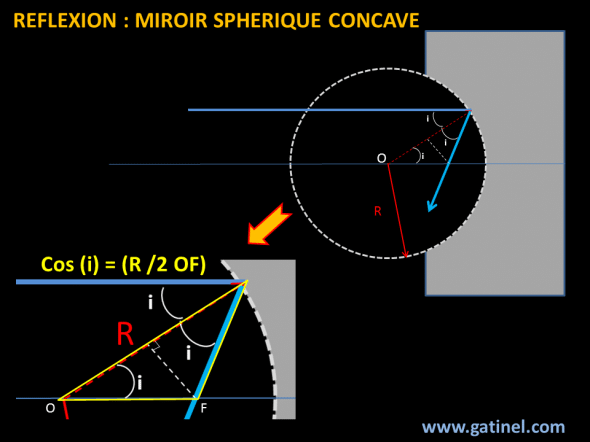When an incident Ray is reflected by a spherical mirror, it forms an angle of incidence i towards the normal to the surface of the mirror. By definition, reflects the angle an angle equal to i to this normal, which passes through the center of curvature of the mirror. The point of intersection with the optical axis (F) allows you to draw an isosceles triangle, including the large (hypotenuse) is OF

We can establish from the figure above the following law:

OF = R / (2 x cos ((i))

The angle i will vary with the distance of the incident Ray to the axis, we deduce from this equality that the position of point F varies with the height of the considered range. The spherical mirror is not a stigmatic system; the image of a point is not a point, except to consider a small beam of incident rays.

When i is small, one can then write: OF = R/2 car cos (i) tends to 1.

For small angles of incidence (the near rays of the optical axis), it can be established that the home of the mirror, where are concentrated the rays, is located at a distance equal to half of the radius of curvature of the mirror. The image formed by the mirror is located in a plan before it, and reverse

## Convex mirror

We can construct an equivalent figure, this time with a convex mirror, and deduce the same equality as before. Geometric construction reveals that the home is located this time "backwards" from the top of the mirror. The position of the image is also located 'back' of the spherical mirror. This should not shock: the image is virtual, but it can quite be 'captured' by the eye, or an image sensor. This means that the rays emitted in reflections "appear to be" plan of image formation (the eye or the sensor cannot know that the rays have undergone prior reflection).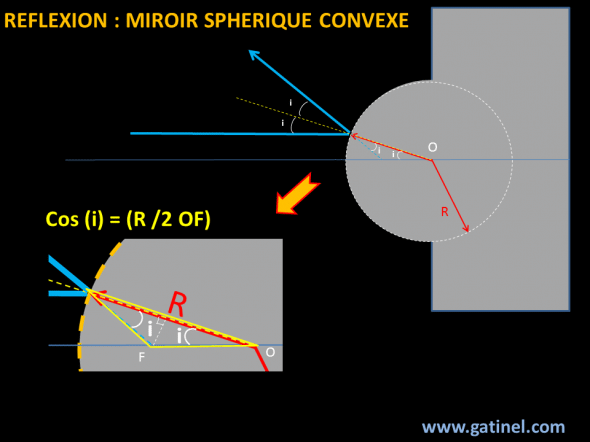When an incident Ray is reflected by a convex spherical mirror, it forms an angle of incidence i towards the normal to the surface of the mirror. By definition, reflects the angle an angle equal to i to this normal, which passes through the center of curvature of the mirror. The point of intersection with the optical axis (F) allows you to draw an isosceles triangle whose side is R.

## Position of the reflected image

The following figure lays the foundations of the problem: we're looking for the position I of the image of a source O point located on the optical axis. With a few approximations on small angles (the tangent of the angle is equal to the angle in radians, and the cosine of a small angle is very close to 1), we can establish a combination formula that calculates the position of the reflected image.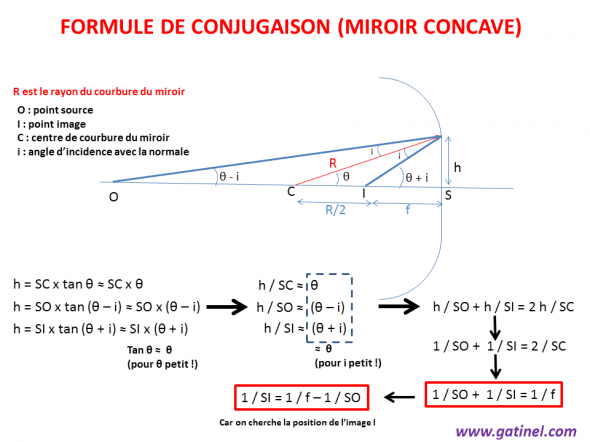On the diagram, O from RADIUS meets the surface of the concave mirror to a height h. It reflects and cut the optical axis in I (attention: I is not exactly located in the distance f, as for a ray incident parallel to the optical axis).

To perform calculations with this formula, it is important to consider the distances as algebraic quantities: to the left from the top, the distances are negative amounts, to the right, positive.

For a convex mirror, the formula is the same, but the sign to the value of the radius of curvature is negative.

It is interesting to note that this formula is similar to the formula of vergence, but that there is no value of refractive index, since the propagation of light occurs in reflection, in the same environment.

These formulas are used to calculate the position of the eye reflections)Purkinje images).

## Example:

We're looking for to know the position of the image of a source object to 40 cm from the surface of the cornea, whose radius of curvature is 8 mm. The home of the mirror is so located in half this distance (4 mm).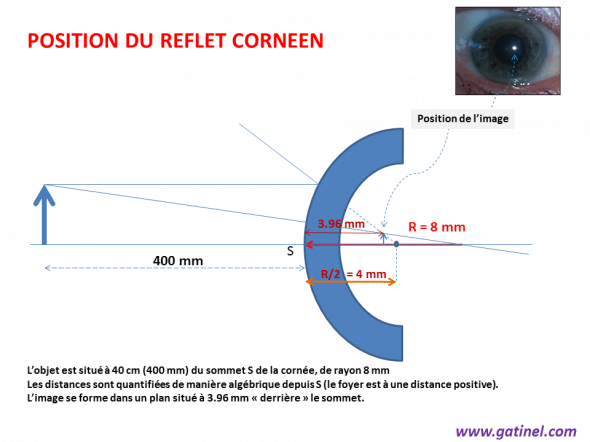Scheme for calculating the position of an image reflected from the cornea (ex: image of a bright light source reflecting on c ^ me corneal)

We can write:

1 = 1, f - 1 /(distance objet) /(distance image)

1 /(distance image) = 1/4 - 1 /-400 = 101/400

Remote image = 400/101 = 3.96 mm

(Virtual) appears in a later plan to the mirror, and the image is not reversed (unlike the case of reflection by a concave mirror - when the object is located before (later) of the household.)